Next: Planetocentric Systems Up: Planetary Positions Previous: Planetary Positions

### Position of Earth and Moon

Tab.5 gives also ecliptic positions of Earth and Moon. DE200 and VSOP87 give only the heliocentric position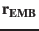of the EMB. DE200 gives in addition the geocentric position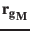of the Moon. If both values are given the position of the Earth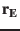can be calculated exactly (within the IAU1976 system) using the mass ratio Moon/Earth of[A. K6] (or its respective value used for the ephemeris) by: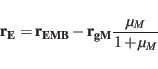(31)

The velocity vector has the same transformation. For the VSOP87 system we apply following formula by J.L.Simon [personal communication] describing the rotation of the Earth around the EMB: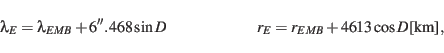(32)

where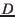is the Delauney argument from eqn.3.5 in Simon et al. (1994):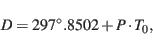(33)

where the rotation period is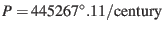. The Earth velocity vector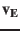can be calculated by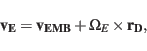(34)

where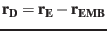and the ecliptic angular velocity vector is given by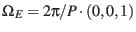.

If onlyandare given the ecliptic position and velocity of the Moon can then be calculated by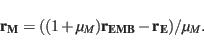(35)

But the precision of the resulting lunar velocity is rather low (190 m/s). Neglecting the difference betweenandincreases the total error in the Earth position to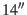(Earth-EMB in Tab.5).

For slightly lower precision without solving the Kepler equation (26) the geometric ecliptic longitude of the Earth can be calculated by the approximation given for the Solar longitude in [A. C24]: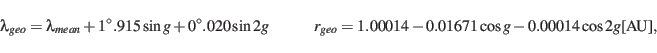(36)

where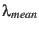and the mean anomaly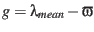for the EMB can be taken from Tab.4. This approximation has also been used by Hapgood (1992). The respective precision is(Earth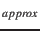in Tab.5).Next: Planetocentric Systems Up: Planetary Positions Previous: Planetary Positions
Markus Fraenz 2017-03-13